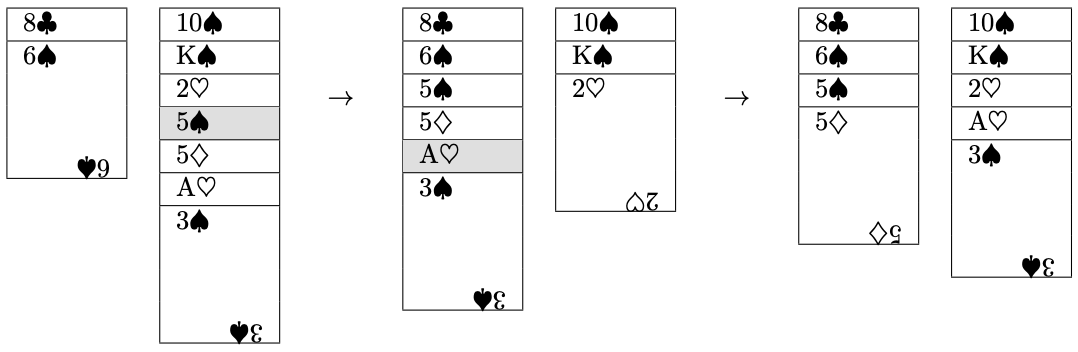시간 제한메모리 제한제출정답맞힌 사람정답 비율
1 초 (추가 시간 없음) 512 MB111100.000%

## 문제

In this problem a variation of <<Scorpion>> solitaire is presented.

You are given a deck of $52$ playing cards which are dealt into seven columns. Every column may have an arbitrary number of the cards, including cases when there are no cards in some columns (we call such columns empty). Each card has a suit ($\diamondsuit$, $\heartsuit$, $\spadesuit$, or $\clubsuit$) and a rank (in increasing order: A, $2$, $3$, $4$, $5$, $6$, $7$, $8$, $9$, $10$, J, Q, K).

On each turn you are allowed to do the following: you choose the current card in some column (you may pick any) and move it onto the bottom card of another column together with all cards on top of it (a bottom part of column is moved as one unit). You are allowed to move the current card only onto a card of the same suit and rank larger exactly by $1$. For example, $5 \spadesuit$ can be moved only onto $6 \spadesuit$, and A$\heartsuit$ can be moved only onto $2 \heartsuit$ as it is shown in picture below. If the current card has rank K, you are allowed to move it only onto an empty column (together with all cards on top of it as well) and only if it lies on an another card (not on the top of a column).The goal of the game is to build $4$ columns of suit sequences from king to ace (K is in top of column, and A is in bottom).

## 입력

You are given $7$ lines, the $i$-th of which describes the $i$-th column. The $i$-th line starts with integer $k_i$ --- the number of cards in the $i$-th column ($0 \le k_i \le 52$), followed by $k_i$ two-symbol strings which describe cards in the $i$-th column from top to bottom. The first symbol encodes a rank ("A", "2", "3", "4", "5", "6", "7", "8", "9", "T", "J", "Q" and "K" for A, $2$, $3$, $4$, $5$, $6$, $7$, $8$, $9$, $10$, J, Q and K correspondingly), the second one encodes a suit ("D", "H", "S" and "C" for $\diamondsuit$, $\heartsuit$, $\spadesuit$ and $\clubsuit$ correspondingly).

It is guaranteed that the input data contains all $52$ cards and that every of them occurs exactly once.

## 출력

If it is impossible to win the game, print "NO". Otherwise, in the first line print "YES", in the second line print the number of moves, and in the third line print cards in order of making turns. If there are several solutions, output any of them.

## 예제 입력 1

14 KD QD JD TD 9D 8D 7D 6D 5D 4D 3D 2D AD KH
12 AS 6C 5C 4C 3C 2C AC 6S 5S 4S 3S 2S
11 KS QS JS TS 9S 8S 7S 5H 4H 3H 2H
1 KC
0
11 8H 7H 6H QC JC TC 9C 8C 7C QH JH
3 AH TH 9H


## 예제 출력 1

YES
10
QH 6C AS KH AH QC 5H 6S TH 8H


## 예제 입력 2

5 JH TH 9H JC AH
2 KH QH
6 6H 2C AC KD 8H 7H
6 QD JD 4H 3H KC QC
10 3S 2S AS 8S 7S 6S 5S 4S QS JS
12 3C TC 9C 8C 7C 6C 5C 4C KS TS 9S 2H
11 TD 9D 8D 7D 6D 5D 4D 3D 2D AD 5H


## 예제 출력 2

YES
20
JH KD 6H KS JC 8H QD KC 2H TS QS 8S 3S AH TC 3C 2C 5H 4H TD